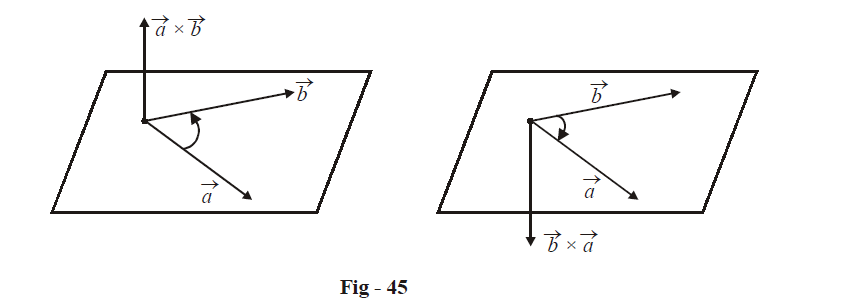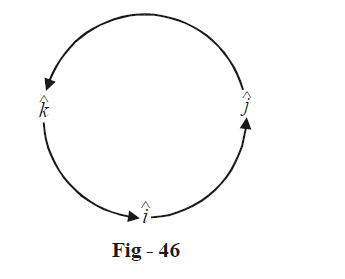# Properties Of Vector Cross Product

Go back to  'Vectors and 3-D Geometry'

We now note some important properties of the cross product:

(i) If $$\vec a\,\,{\text{and}}\,\,\vec b$$ are parallel, their cross product is zero, i.e.

$\vec a \times \vec b = \vec 0$

since $$\sin \theta = 0$$. Conversely, if $$\vec a \times \vec b = \vec 0,$$ then $$\vec a\,\,{\text{and}}\,\,\vec b$$ must be parallel.

(ii) The cross product is not commutative. In fact,

$\vec b \times \vec a = - \vec a \times \vec b$

This is because the direction of $$\vec a \times \vec b$$ was defined so that $$\vec a$$ ,$$\vec b$$ and $$\vec a \times \vec b$$ form a right handed system(iii) The cross product is distributive over vector addition:

\begin{align}&\quad\qquad \vec a \times (\vec b + \vec c) = \vec a \times \vec b + \vec a \times \vec c \hfill \\\\&and\quad(\vec a + \vec b) \times \vec c\; = \vec a \times \vec c + \vec b \times \vec c \hfill \\ \end{align}

(iv)$$\hat i \times \hat i = \hat j \times \hat j = \hat k \times \hat k = \vec 0$$

$$\hat i \times \hat j = \hat k,\;\;\hat j \times \hat k = \hat i,\;\;\hat k \times \hat i = \hat j$$

These relations can be remembered asGoing in the reverse direction, we have

$\hat j \times \hat i = - \hat k,\;\;\hat i \times \hat k = - \hat j,\;\;\hat k \times \hat j = - \hat i$

Thus, for two vectors $$\vec a = {a_1}\hat i + {a_2}\hat j + {a_3}\hat k\,\,and\,\,\vec b = {b_1}\hat i + {b_2}\hat j + {b_3}\hat k$$  we have

\begin{align}&\vec a \times \vec b \,= ({a_1}\hat i + {a_2}\hat j + {a_3}\hat k) \times ({b_1}\hat i + {b_2}\hat j + {b_3}\hat k) \hfill \\\\&\qquad\;= {a_1}\;{b_2}\hat k - {a_1}{b_3}\hat j - {a_2}{b_1}\hat k + {a_2}{b_3}\hat i + {a_3}{b_1}\hat j - {a_3}{b_2}\hat i \hfill \\\\&\qquad\; = \hat i({a_2}{b_3} - {a_3}{b_2}) + \hat j({a_3}{b_1} - {a_1}{b_3}) + \hat k({a_1}{b_2} - {a_2}{b_1}) \hfill \\ \end{align}

This can be written concisely in determinant notation as

\vec a \times \vec b = \left| {\begin{align}&{\hat i}&{\hat j}&&{\hat k} \\& {{a_1}}&{{a_2}}&&{{a_3}} \\ & {{b_1}}&{{b_2}}&&{{b_3}} \end{align}} \right|

(v) The unit vector(s) $$\hat r$$ normal to the plane of $$\vec a\,\,{\text{and}}\,\,\vec b$$ can be written as

$\hat r = \pm \frac{{\vec a \times \vec b}}{{\left| {\vec a \times \vec b} \right|}}$

Vectors
grade 11 | Questions Set 1
Vectors